How to make a fraction in Word (type, input with shortcut and insert with field, tacked and skewed)

Lionsure 2020-11-06 Original by the website

There are three ways to type fractions in Word, one is to use Equation, the second is to use field; the latter can be divided into using shortcut keys and insert field. There are four types of fractions using Equations, namely stacked, skewed, linear and small fractions, but the fraction needs to have a Equation input box; and input with field does not have a Equation input box. Using shortcut keys to type fractions is achieved by inserting field codes.

Sometimes the inputted fractions are not displayed completely. This is usually caused by too small line spacing. Just increase the line spacing appropriately and the fractions will be displayed completely. The following are specific examples of how to type fractions in Word (i.e. how to create a fraction in Word).

I. Method 1 of how to make a fraction in Word: Use Equation to input (used in Word 2007 and above, how to insert fraction in Word)

1. Position the cursor where you want to type a fraction, select the "Insert" tab, click the "Equation" in the upper right corner of screen (or press the shortcut key Alt + =), then a Equation input box is inserted into the document, and the "Design" tab is automatically displayed on the right or "View" tab and select it, click "Fraction", select the first one in the pop-up style, and insert a Equation into the Equation input box; select the numerator box and enter 3, then check the denominator box and enter 5, then enter the stacked fraction 3/5. Enter an skewed fraction 2/7 in the same way; the operation process steps are shown in Figure 1: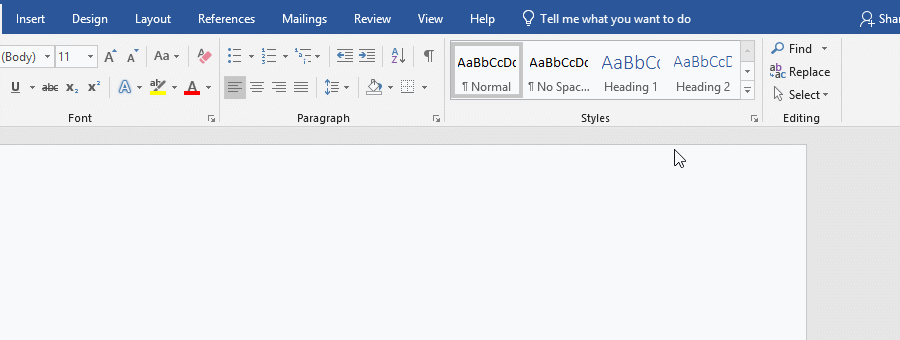Figure 1

There are four types of fractions in Word. In addition to the stacked and skewed types entered above, there are also linear and small fractions; if you want to use the latter two fractional styles, just choose them using the method above.

2. Adjust the alignment. Click after 2/7 to display the Equation input box, click the arrow down in the lower right corner of it, and select "Justification" → Left in the pop-up options, then the two fractions will be aligned to the left. The operation process steps are shown in Figure 2: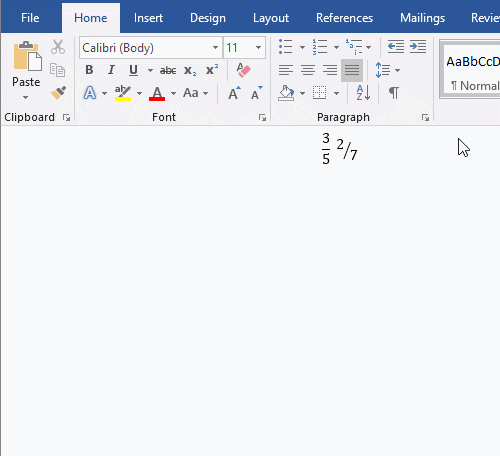Figure 2

II.  Method 2 of how to make a fraction in Word: Input by field

(I) How to input fraction in Word Using shortcut keys

1. Position the cursor to the position where you want to input a fraction, press Ctrl + F9, a pair of braces will appear, copy "EQ \F" into the braces (you can also enter), then enter (2,3), press Shift + F9, then enter 2/3; the operation process steps are shown in Figure 3: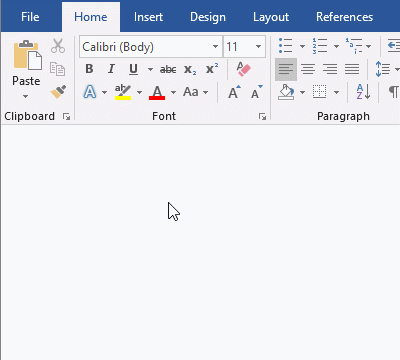Figure 3

2. Note: After entering the field code {EQ \F(2,3) }, you can also right-click them and select "Toggle Field Codes" in the pop-up menu, and the Field Codes will be converted to a fraction; then right-click the fraction and also select "Toggle Field Codes", the fraction is converted to a field code; the steps of the operation process are shown in Figure 4: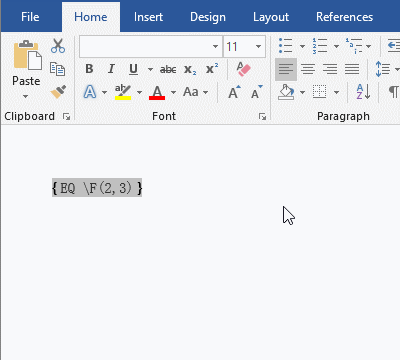Figure 4

(II) How to enter fractions in Word with the insert field

Word 2019:

Position the cursor where you want to enter the fraction, select the "Insert" tab, click "Quick Parts", and select "Field" from the pop-up menu, open the "Field" dialog box; click the "Categories" drop-down list box, then select "Equations and Formulas", and then select "Eq" under "Field names"; enter \F(1,6) on the right of "Eq", click "OK", enter 1/6; the procedure steps, as shown in Figure 5: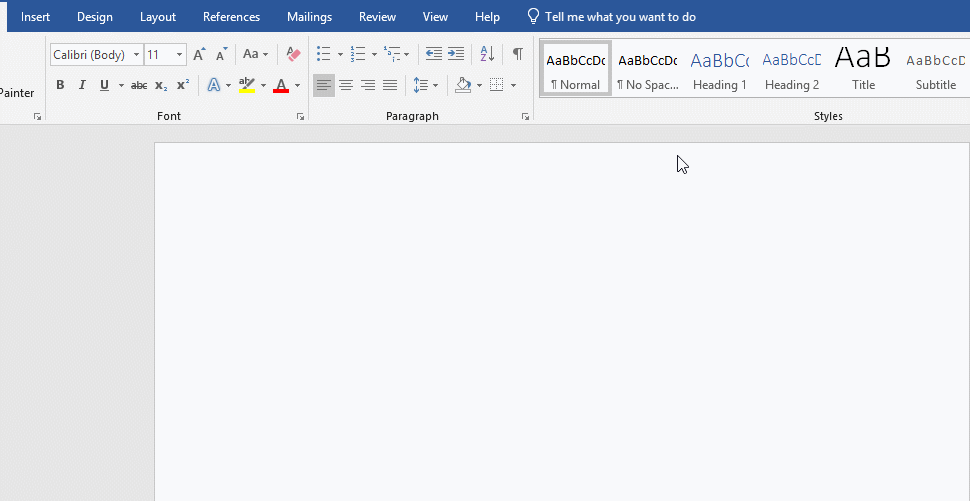Figure 5

Word 2016 and before:

Position the cursor where you want to enter the fraction, select the "Insert" tab, click "Quick Parts", and select "Field" from the pop-up menu (click "Field" directly in Word 2003), open the "Field" dialog box; click the "Categories" drop-down list box, then select "Equations and Formulas", and then select "Eq" under "Field names"; click "Field Code" in the lower left corner of window, and then click "Options", open the "Field Options" dialog box, double-click \F(,) under "Switch", it will be filled into the "Field code: EQ" input box, enter the numerator 1 on the left of comma in the brackets, and enter the denominator 6 on the right, and click "OK", return to the "Field" dialog box, and then click "OK", the field codes {EQ \F(1,6)} will be inserted into the document, press Shift + F9, then enter 1/6.

III. Word fraction display is not complete

The fraction that is completely displayed is usually caused by too small line spacing in Word. Just increase the line spacing and it will be fully displayed. The method is: Position the cursor in the text (select the text) to be adjusted, the current tab is "Home", and click "Line and Paragraph Spacing" icon, select 1.0, then the fraction will be displayed completely. The operation process steps are shown in Figure 6: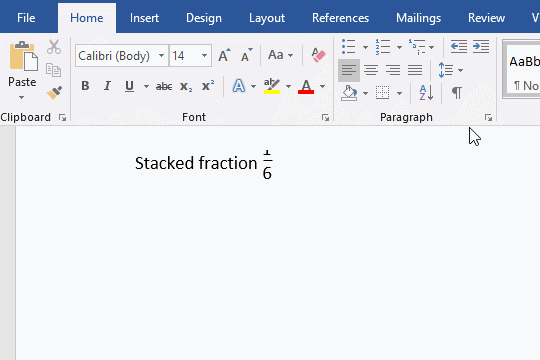Figure 6